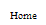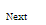# C Programming language

Operators in C
 Previous Home Next

## Assignment Operator(=)

The assignment operator assigns a value to a variable. There is two side in assignment operator :

1. In assignment operator (=) left side value known as the lvalue (left value) contain variable.
2. In assignment operator (=) right side value known as rvalue (right value) contain the value of that variable.

The in assignment operator (=) is variable has in lvalue and the value of that variable is depend in to the rvalue which may be either a variable, constant. The result of assignment operation any combination of these.

```
int a=10;

```

This statement assigns the integer value 10 to the variable a.

Arithmetic operators ( +, -, *, /, % )

The five arithmetical operations supported by the C language are:

 Sign Name + addition - subtraction * multiplication / division % modulo
Compound assignment (+=, -=, *=, /=, %=, >>=, <<=, &=, ^=, |=)

when want to change the current stored value of a variable by performing an operation we can use compoundassignment operators:

 Expression Evaluation value += increase; value = value + increase; a -= 5; a = a - 5; a /= b; a = a / b; price *= units + 1; price = price * (units + 1)

### Example

```
nt main ()
{
int a, b=3;
a = b;
a+=10;// equivalent to a=a+10
printf("\n%d",a );
return 0;
}

```
Increase and decrease (++, --)

Shortening even more some expressions, the increase operator (++) and the decrease operator (--) increase

or

Reduce by one the value stored in a variable. They are equivalent to +=1 and to -=1, respectively. Thus:

 1 a++; 2 a+=1; 3 a=+1;
Relational and equality operators ( ==, !=, >, < >=, <= )

Between two expressions if evaluated for comparison C language provide relational and equality operators. The result of a relational operation is a Boolean value when compare two expression that can only be true or false, according to its Boolean result.

 Sign Name == Equal to != Not equal to > Greater than < Less than >= Greater than or equal to <= Less than or equal to
Logical Operator( && ,||,!)

### && OPERATOR

In this when both conditions true then the result is true other wise in each condition the result will be false.

 a b a && b true true true true false false false true false false false false

### || OPERATOR

In this when both condition false then result false otherwise in each condition the result is true.

 a b a || b true true true true false true false true true false false false
Conditional operator ( ? :)

The conditional operator evaluates an expression if the condition is true then return true value otherwise return the false value according to condition.

```
condition ? true: false
// conditional operator
int main ()
{
int a,b,c;
a=10;
b=20;
c = (a>b) ? a : b;
printf("\n%d",c );
return 0;
}

```

In this example a was 10and b was 20, so the expression being evaluated (a>b) was not true, thus the first value specified after the question mark was discarded in favor of the second value (the one after the colon) which was b, with a value of 20.

[an error occurred while processing this directive]

Operators

Assignment  Operator(=)

### The assignment operator assigns a value to a variable. There is two side in assignment operator :

• In assignment operator (=) left side value  known as the lvalue (left value)  contain variable.

• In assignment operator (=)  right side value known as rvalue (right value) contain the value of that variable.

The  in assignment operator (=) is variable has in lvalue and the value of that variable is depend in to the rvalue  which  may be either a variable, constant. The result of assignment operation any combination of these.

int a=10;

This statement assigns the integer value 10 to the variable
a.

Arithmetic operators ( +, -, *, /, % )

The five arithmetical operations supported by the C language are:

 + addition - subtraction * multiplication / division % modulo

### Compound assignment (+=, -=, *=, /=, %=, >>=, <<=, &=, ^=, |=)

when want to change the current stored value of a variable by performing an operation we can use compound
assignment operators:

expression

evaluation

value += increase;

value = value + increase;

a -= 5;

a = a - 5;

a /= b;

a = a / b;

price *= units + 1;

price = price * (units + 1)

Example:

int main ()
{
int a, b=3;

a = b;
a+=10;// equivalent to a=a+10
printf("\n%d",a );

return 0;
}

### Increase and decrease (++, --)

Shortening even more some expressions, the increase operator (++) and the decrease operator (--) increase or
reduce by one the value stored in a variable. They are equivalent to
+=1 and to -=1, respectively. Thus:

 1 a++; 2 a+=1; 3 a=+1;

### Relational and equality operators ( ==, !=, >, < >=, <= )

Between two expressions if evaluated for comparison C language provide relational and equality operators. The
result of a relational operation is a Boolean value  when compare two expression  that can only be true or false,
according to its Boolean result.

 == Equal to != Not equal to > Greater than < Less than >= Greater than or equal to <= Less than or equal to

Logical Operator( && ,||,!)

&& OPERATOR

In this when both conditions true then the result is true other wise in each condition the result will be false.

a

b

a && b

true

true

true

true

false

false

false

true

false

false

false

false

```

```
|| OPERATOR

In this when both condition false then result false otherwise in each condition the result is true.

a

b

a || b

true

true

true

true

false

true

false

true

true

false

false

false

### Conditional operator ( ? :)

The conditional operator evaluates an expression if the condition is true then return true value otherwise return the
false value according to condition.

condition ? true: false

// conditional operator

int main ()

{

int a,b,c;

a=10;

b=20;

c = (a>b) ? a : b;

printf("\n%d",c );

return 0;

}

In this example a was 10and b was 20, so the expression being evaluated (a>b) was not true, thus the first value specified after the question mark was discarded in favor of the second value (the one after the colon) which was b, with a value of 20.
Comma operator ( , )

The comma operator (,) is used to separate two or more expressions where only one expression is expected. When
the set of expressions has to be evaluated for a value, only the rightmost expression is considered.

For example, the following code:

 ``a = (b=30, b+20);``

Would first assign the value 30 to b, and then assign b+20 to variable a. So, at the end, variable a would contain the value 50 while variable b would contain value 30.

Bitwise Operators ( &, |, ^, ~, <<, >> )

Bitwise operators modify variables or shifting the bit left or right patterns that represent the values they store.

operator

asm equivalent

description

&

AND

Bitwise AND

|

OR

Bitwise Inclusive OR

^

XOR

Bitwise Exclusive OR

~

NOT

Unary complement (bit inversion)

<<

SHL

Shift Left

>>

SHR

Shift Right

### sizeof()

This operator will accept one parameter, which can be a variable itself and returns the size of it in bytes of that type or object.

 ``a = sizeof (char);``

This will assign the value 1 to a because char is a one-byte long type. The value returned by sizeof is a constant, so it is always determined before program execution.R4RCC TutorialsC Basic Tutorials1.4Operators
 Previous Home Next